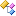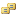﻿FinAnalysis.Predicates Namespace

# FinAnalysis.Predicates Namespace

The FinAnalysis.Listeners namespace contains classes for predicates.Classes
ClassDescriptionBarClose
Returns closing price of the bar.BarHigh
Returns highest price of the bar.BarLow
Returns lowest price of the bar.BarOpen
Returns opening price of the bar.BarVolume
Returns volume of the bar.Constant
Returns constant value.Count
Calculates number of times the signal was generated during the given period.Difference
Calculates difference of two series.Distance
Calculates distance between two series.IsAbove
Generates signal when the first line is above the second line during the given period.IsApproachingAbove
Generates signal when time series is approaching from above to the given level.IsApproachingBelow
Generates signal when time series is approaching from below to the given level.IsBelow
Generates signal when the first line is below the second line during the given period.IsConverging
Generates singal when distance between two series is decreasing.IsCrossDown
Generates singal when the first line intersects the second line from up to downIsCrossUp
Generates singal when the first line intersects the second line from down to up.IsDecreasing
IsDecreasing predicate.IsDiverging
Generates singal when distance between two series is increasing.IsDropped
Generates signal the value dropped by given amount of percents during the period.IsEqual
Generates a signal when the values of two given time series are equal with given precision during the given interval.IsGained
Generates signal the value gained by given amount of percents during the period.IsGreater
IsGreater predicate.IsIncreasing
IsIncreasing predicate.IsInInterval
IsInInterval predicate.IsLess
IsLess predicate.IsNear
Generates signal when relative distance between two lines are smaller than given level (default : 2%).IsNewHigh
Generates signal when the maximum value is achieved on the short period.IsNewLow
Generates signal when the minimum value is achieved on the short period.IsTime
Generates signal when current time satisfies the given condition.LogicalAnd
Logical AND.LogicalAndNot
Logical AND NOT.LogicalNot
Logical NOT.LogicalOr
Logical OR.LogicalOrNot
Logical OR NOT.LogicalXor
Logical XOR (exclusive OR).LogicalXorNot
Logical XOR NOT (exclusive OR with negation second argument).LogReturn
Computes logarithmic rate of return.Product
Calculates product of two series.Ratio
Calculates quotient of two series.Return
Computes arithmetic rate of return.Sum
Calculates sum of two series.WeightedSum
Calculates weighted sum of two series.Enumerations
EnumerationDescriptionIsTimeComparisonOperator
Comparison operator type.